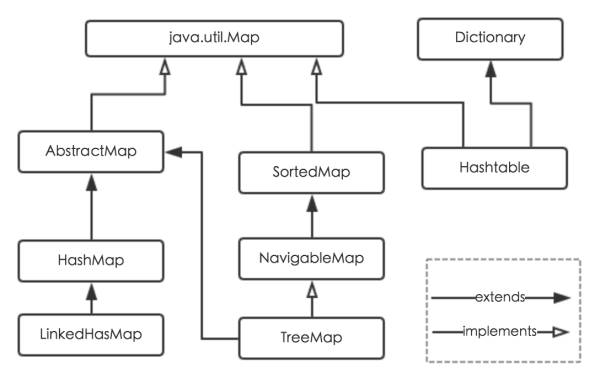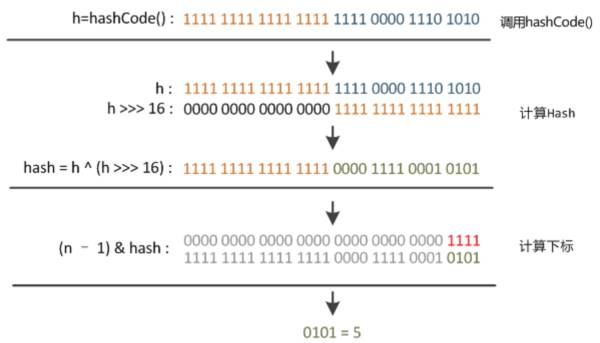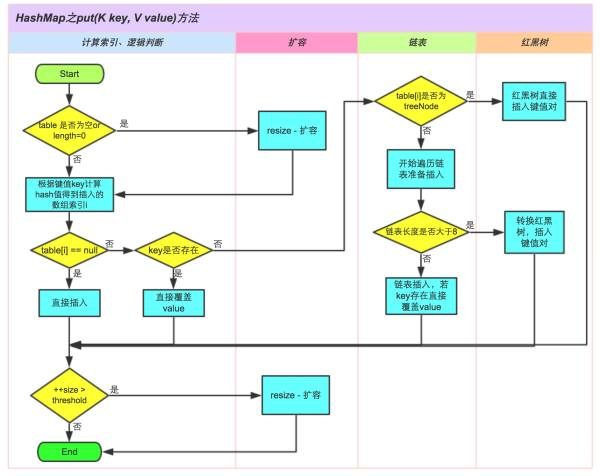# HashMap详解

JDK1.8对HashMap底层的实现进行了优化，例如引入红黑树的数据结构和扩容的优化等。

Java为数据结构中的映射定义了一个接口java.util.Map1、HashMap：它根据键的hashCode值存储数据，大多数情况下可以直接定位到它的值，因而具有很快的访问速度。

HashMap最多只允许一条记录的键为null，允许多条记录的值为null。非线程安全。

2、Hashtable：Hashtable是遗留类，很多映射的常用功能与HashMap类似，不同的是它承自Dictionary类。线程安全。并发性不如ConcurrentHashMap，因为ConcurrentHashMap引入了分段锁。

4、TreeMap：TreeMap实现SortedMap接口，能够把它保存的记录根据键排序，默认是按键值的升序排序，也可以指定排序的比较器，当用Iterator遍历TreeMap时，得到的记录是排过序的。

（1） 存储结构-字段
（2） 功能实现-方法

HashMap是数组+链表+红黑树（JDK1.8增加了红黑树部分）实现的。HashMap类中有一个非常重要的字段，就是 Node[] table，即哈希桶数组

static class Node<K,V> implements Map.Entry<K,V> {   final int hash;    //用来定位数组索引位置   final K key;   V value;   Node<K,V> next;   //链表的下一个node   Node(int hash, K key, V value, Node<K,V> next) { ... }   public final K getKey(){ ... }   public final V getValue() { ... }   public final String toString() { ... }   public final int hashCode() { ... }   public final V setValue(V newValue) { ... }   public final boolean equals(Object o) { ... }}

Node是HashMap的一个内部类，实现了Map.Entry接口，本质是就是一个映射(键值对)。上图中的每个黑色圆点就是一个Node对象。

HashMap就是使用哈希表来存储的。Java中HashMap采用了拉链法解决冲突。

map.put("美团","小美");

HashMap的默认构造函数就是对下面几个字段进行初始化

int threshold;             // 所能容纳的key-value对极限 final float loadFactor;    // 负载因子int modCount;              // 用来记录HashMap内部结构发生变化的次数int size;

HashMap采用这种非常规设计，主要是为了在取模和扩容时做优化，同时为了减少冲突，HashMap定位哈希桶索引位置时，也加入了高位参与运算的过程。

HashMap的内部功能实现很多，本文主要讲述：

1、根据key获取哈希桶数组索引位置

2、put方法的详细执行

3、扩容过程

方法一：static final int hash(Object key) {   //jdk1.8 & jdk1.7    int h;    // h = key.hashCode() 为第一步 取hashCode值    // h ^ (h >>> 16)  为第二步 高位参与运算    return (key == null) ? 0 : (h = key.hashCode()) ^ (h >>> 16);}方法二：static int indexFor(int h, int length) {  //jdk1.7的源码，jdk1.8没有这个方法，但是实现原理一样的    return h & (length-1);  //第三步 取模运算}JDK1.8HashMap的put方法源码如下:

public V put(K key, V value) {   // 对key的hashCode()做hash   return putVal(hash(key), key, value, false, true); } final V putVal(int hash, K key, V value, boolean onlyIfAbsent, boolean evict) {   Node<K, V>[] tab;   Node<K, V> p;   int n, i;   // 步骤①：tab为空则创建   if ((tab = table) == null || (n = tab.length) == 0)     n = (tab = resize()).length;   // 步骤②：计算index，并对null做处理   if ((p = tab[i = (n - 1) & hash]) == null)     tab[i] = newNode(hash, key, value, null);   else {     Node<K, V> e;     K k;     // 步骤③：节点key存在，直接覆盖value     if (p.hash == hash && ((k = p.key) == key || (key != null && key.equals(k))))       e = p;     // 步骤④：判断该链为红黑树     else if (p instanceof TreeNode)       e = ((TreeNode<K, V>) p).putTreeVal(this, tab, hash, key, value);     // 步骤⑤：该链为链表     else {       for (int binCount = 0;; ++binCount) {         if ((e = p.next) == null) {           p.next = newNode(hash, key, value, null);           // 链表长度大于8转换为红黑树进行处理           if (binCount >= TREEIFY_THRESHOLD - 1) // -1 for 1st             treeifyBin(tab, hash);           break;         }         // key已经存在直接覆盖value         if (e.hash == hash && ((k = e.key) == key || (key != null && key.equals(k))))           break;         p = e;       }     }     if (e != null) { // existing mapping for key       V oldValue = e.value;       if (!onlyIfAbsent || oldValue == null)         e.value = value;       afterNodeAccess(e);       return oldValue;     }   }   ++modCount;   // 步骤⑥：超过最大容量 就扩容   if (++size > threshold)     resize();   afterNodeInsertion(evict);   return null; }

void resize(int newCapacity) {   //传入新的容量   Entry[] oldTable = table;    //引用扩容前的Entry数组   int oldCapacity = oldTable.length;            if (oldCapacity == MAXIMUM_CAPACITY) {  //扩容前的数组大小如果已经达到最大(2^30)了       threshold = Integer.MAX_VALUE; //修改阈值为int的最大值(2^31-1)，这样以后就不会扩容了       return;   }    Entry[] newTable = new Entry[newCapacity];  //初始化一个新的Entry数组  transfer(newTable);                         //！！将数据转移到新的Entry数组里  table = newTable;                           //HashMap的table属性引用新的Entry数组  threshold = (int)(newCapacity * loadFactor);//修改阈值  }

transfer()方法将原有Entry数组的元素拷贝到新的Entry数组里。

void transfer(Entry[] newTable) {   Entry[] src = table;                   //src引用了旧的Entry数组   int newCapacity = newTable.length;   for (int j = 0; j < src.length; j++) { //遍历旧的Entry数组       Entry<K,V> e = src[j];             //取得旧Entry数组的每个元素       if (e != null) {           src[j] = null;//释放旧Entry数组的对象引用（for循环后，旧的Entry数组不再引用任何对象）           do {               Entry<K,V> next = e.next;               int i = indexFor(e.hash, newCapacity); //！！重新计算每个元素在数组中的位置               e.next = newTable[i]; //标记               newTable[i] = e;      //将元素放在数组上               e = next;             //访问下一个Entry链上的元素           } while (e != null);       }   }}

HashMap在多线程的情况下可能链结构会受到破坏，导致无限循坏(JDK8 可能已经解决)

(1) 扩容是一个特别耗性能的操作，所以当程序员在使用HashMap的时候，估算map的大小，初始化的时候给一个大致的数值，避免map进行频繁的扩容。

(2) 负载因子是可以修改的，也可以大于1，但是建议不要轻易修改，除非情况非常特殊。

(3) HashMap是线程不安全的，不要在并发的环境中同时操作HashMap，建议使用ConcurrentHashMap。

(4) JDK1.8引入红黑树大程度优化了HashMap的性能。

1. 美团点评技术团队 Java 8系列之重新认识HashMap https://zhuanlan.zhihu.com/p/21673805

2. 为什么一般hashtable的桶数会取一个素数 http://blog.csdn.net/liuqiyao_01/article/details/14475159

posted @ 2018-06-07 19:15  南望孤笑  阅读(2237)  评论(0编辑  收藏  举报